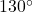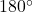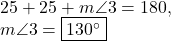## Three angles on a straight line. The first and second angles each measures 25°. What does the third angle measure?

Question

Three angles on a straight line. The first and second angles each measures 25°. What does the third angle measure?

in progress 0
2 months 2021-08-28T08:09:56+00:00 2 Answers 5 views 0

130

Step-by-step explanation:

A straight like measures 180°

25+25=50

180-50=130

plz mark brainliest 🙂A straight line can denote half a circle, each side having. Therefore, the sum of these three angles should be. Since the first two angles both measure, we can set up the following equation and solve:.